### Home > CCA2 > Chapter Ch11 > Lesson 11.2.3 > Problem11-71

11-71.
1.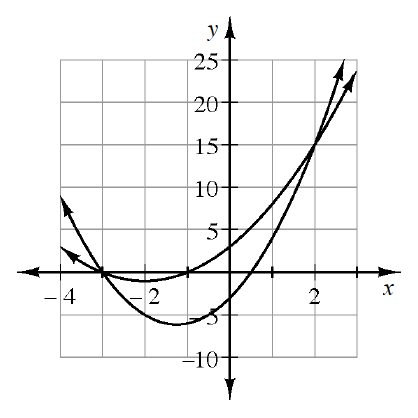The graphs of f(x) = 2x2 + 5x − 3 and g(x) = x2 + 4x + 3 are shown at right. Use the graphs to solve: Homework Help ✎

1. f(x) = g(x)

2. f(x) > g(x)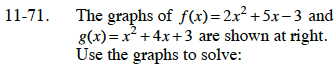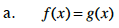Look at the graph. At which points do the two functions intersect?

You can check you answer by setting the two equations equal to each other and solving for the possible values of x.

x = −3 or x = 2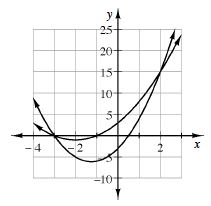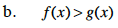At what points, or sections, are the y-values for f(x) greater than those for g(x)?